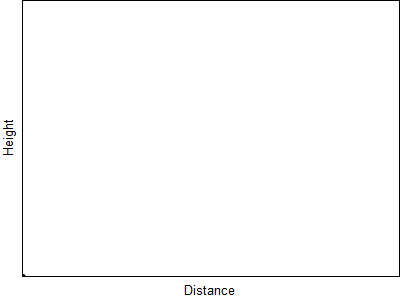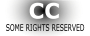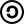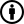#### You may also like### Ball Bearings

If a is the radius of the axle, b the radius of each ball-bearing, and c the radius of the hub, why does the number of ball bearings n determine the ratio c/a? Find a formula for c/a in terms of n.### Overarch 2

Bricks are 20cm long and 10cm high. How high could an arch be built without mortar on a flat horizontal surface, to overhang by 1 metre? How big an overhang is it possible to make like this?### Cushion Ball

The shortest path between any two points on a snooker table is the straight line between them but what if the ball must bounce off one wall, or 2 walls, or 3 walls?

# Model Solutions

### Extra information needed

Whether the shot-put always has the same initial speed or if it depends on its mass, and if so what the masses of the shots in those scenarios where it is not stated are (affects situations 4 and 6).
More details of the wind conditions - whether it is to be assumed it is of constant speed and direction, or if the velocity varies depending on position and if so (affects 6).

### Order

Without this information, the following order for the range of the shot-put in the different situations can be established:
5 (greatest)
1
2
3 (least)

The following is a brief qualitative description of how the various factors affect the range or the shot-put, for a more in depth numerical analysis see the Modelling Assumptions article.

#### Variable g

Gravitational force follows an inverse square relationship with separation, therefore assuming constant g is defined as that at ground level, a object subject to variable g will travel very slightly further than one under constant g (and all other conditions the same) as the accelerations due to gravity during its motion will be slightly lower. Over the heights that could be achievably reached by a shot-put in reality, the difference would be so small it would be impossible to measure.

#### Air resistance

Air resistance always acts to oppose motion and so will slow both the horizontal and vertical speeds of the shot-put, at the velocities likely to be encountered here the drag force being modelled as proportional to the relative velocity between the object and fluid (Stokes' drag). Although the actual mathematics involved in showing how this will affect the overall range is quite complicated (see Modelling article for details), intutition and real-world experience suggest that including air resistance will decrease range and that the more viscous the air conditions (e.g. misty vs. dry) the greater the reduction in range will be.

Below is an animation showing how Stokes' drag affects the modelled trajectory of a projected object.###### Released under Creative Commons Attribution Share Alike 3.0 Licence Author: AllenMcC
Both objects are thrown at the same angle and with the same initial velocity. The blue object doesn't experience any drag and moves along a parabola. The black object experiences air resistance (Stokes' drag).

#### Wind

A simple way of considering the effect of wind is in terms of how it affects the air resistance force. Here air resistance is being modelled as proportional in magnitude to (and opposite in direction to) the relative velocity between the shot-put and the fluid it is moving through. If the fluid is moving (wind) this will affect the relative velocity and so also the drag force.

If we consider only cases where the wind velocity is along the line of the shot-put's horizontal velocity (with respect to the ground) then if the wind is acting in the same direction as the shot-put's motion, the relative velocity between fluid and shot will decrease and so correspondingly the drag force and retardation of motion. If the wind speed is greater than that of the shot, the drag force will actually assist the motion of the shot (with respect to the ground). Conversely if the wind is opposing the shot's motion it will increase the relative velocity and so also the drag force.